Next: Impedance Matching Up: Inductance Previous: The Circuit

## Transformers

A transformer is a device for stepping-up, or stepping-down, the voltage of an alternating electric signal. Without efficient transformers, the transmission and distribution of AC electric power over long distances would be impossible. Figure 51 shows the circuit diagram of a typical transformer. There are two circuits. Namely, the primary circuit, and the secondary circuit. There is no direct electrical connection between the two circuits, but each circuit contains a coil which links it inductively to the other circuit. In real transformers, the two coils are wound onto the same iron core. The purpose of the iron core is to channel the magnetic flux generated by the current flowing around the primary coil, so that as much of it as possible also links the secondary coil. The common magnetic flux linking the two coils is conventionally denoted in circuit diagrams by a number of parallel straight lines drawn between the coils.Let us consider a particularly simple transformer in which the primary and secondary coils are solenoids sharing the same air-filled core. Suppose that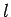is the length of the core, andis its cross-sectional area. Letbe the total number of turns in the primary coil, and let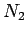be the total number of turns in the secondary coil. Suppose that an alternating voltage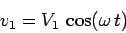(281)

is fed into the primary circuit from some external AC power source. Here,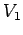is the peak voltage in the primary circuit, andis the alternation frequency (in radians per second). The current driven around the primary circuit is written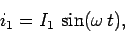(282)

whereis the peak current. This current generates a changing magnetic flux, in the core of the solenoid, which links the secondary coil, and, thereby, inductively generates the alternating emf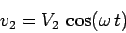(283)

in the secondary circuit, whereis the peak voltage. Suppose that this emf drives an alternating current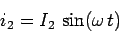(284)

around the secondary circuit, where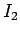is the peak current.

The circuit equation for the primary circuit is written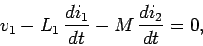(285)

assuming that there is negligible resistance in this circuit. The first term in the above equation is the externally generated emf. The second term is the back-emf due to the self inductance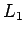of the primary coil. The final term is the emf due to the mutual inductance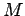of the primary and secondary coils. In the absence of any significant resistance in the primary circuit, these three emfs must add up to zero. Equations (281), (282), (284), and (285) can be combined to give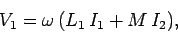(286)

since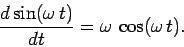(287)

The alternating emf generated in the secondary circuit consists of the emf generated by the self inductance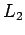of the secondary coil, plus the emf generated by the mutual inductance of the primary and secondary coils. Thus,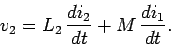(288)

Equations (282), (283), (284), (287), and (288) yield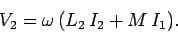(289)

Now, the instantaneous power output of the external AC power source which drives the primary circuit is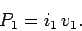(290)

Likewise, the instantaneous electrical energy per unit time transfered inductively from the primary to the secondary circuit is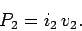(291)

If resistive losses in the primary and secondary circuits are negligible, as is assumed to be the case, then, by energy conservation, these two powers must equal one another at all times. Thus,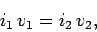(292)

which easily reduces to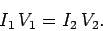(293)

Equations (286), (289), and (293) yield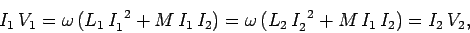(294)

which gives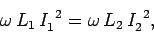(295)

and, hence,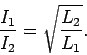(296)

Equations (293) and (296) can be combined to give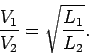(297)

Note that, although the mutual inductance of the two coils is entirely responsible for the transfer of energy between the primary and secondary circuits, it is the self inductances of the two coils which determine the ratio of the peak voltages and peak currents in these circuits.

Now, from Sect. 10.2, the self inductances of the primary and secondary coils are given by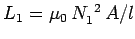and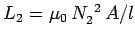, respectively. It follows that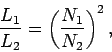(298)

and, hence, that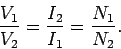(299)

In other words, the ratio of the peak voltages and peak currents in the primary and secondary circuits is determined by the ratio of the number of turns in the primary and secondary coils. This latter ratio is usually called the turns-ratio of the transformer. If the secondary coil contains more turns than the primary coil then the peak voltage in the secondary circuit exceeds that in the primary circuit. This type of transformer is called a step-up transformer, because it steps up the voltage of an AC signal. Note that in a step-up transformer the peak current in the secondary circuit is less than the peak current in the primary circuit (as must be the case if energy is to be conserved). Thus, a step-up transformer actually steps down the current. Likewise, if the secondary coil contains less turns than the primary coil then the peak voltage in the secondary circuit is less than that in the primary circuit. This type of transformer is called a step-down transformer. Note that a step-down transformer actually steps up the current (i.e., the peak current in the secondary circuit exceeds that in the primary circuit).

AC electricity is generated in power stations at a fairly low peak voltage (i.e., something like 440V), and is consumed by the domestic user at a peak voltage of 110V (in the U.S.). However, AC electricity is transmitted from the power station to the location where it is consumed at a very high peak voltage (typically 50kV). In fact, as soon as an AC signal comes out of a generator in a power station it is fed into a step-up transformer which boosts its peak voltage from a few hundred volts to many tens of kilovolts. The output from the step-up transformer is fed into a high tension transmission line, which typically transports the electricity over many tens of kilometers, and, once the electricity has reached its point of consumption, it is fed through a series of step-down transformers until, by the time it emerges from a domestic power socket, its peak voltage is only 110V. But, if AC electricity is both generated and consumed at comparatively low peak voltages, why go to the trouble of stepping up the peak voltage to a very high value at the power station, and then stepping down the voltage again once the electricity has reached its point of consumption? Why not generate, transmit, and distribute the electricity at a peak voltage of 110V? Well, consider an electric power line which transmits a peak electric power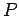between a power station and a city. We can think of, which depends on the number of consumers in the city, and the nature of the electrical devices which they operate, as essentially a fixed number. Suppose thatand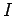are the peak voltage and peak current of the AC signal transmitted along the line, respectively. We can think of these numbers as being variable, since we can change them using a transformer. However, since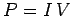, the product of the peak voltage and the peak current must remain constant. Suppose that the resistance of the line is. The peak rate at which electrical energy is lost due to ohmic heating in the line is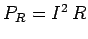, which can be written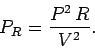(300)

Thus, if the powertransmitted down the line is a fixed quantity, as is the resistanceof the line, then the power lost in the line due to ohmic heating varies like the inverse square of the peak voltage in the line. It turns out that even at very high voltages, such as 50kV, the ohmic power losses in transmission lines which run over tens of kilometers can amount to up to 20% of the transmitted power. It can readily be appreciated that if an attempt were made to transmit AC electric power at a peak voltage of 110V then the ohmic losses would be so severe that virtually none of the power would reach its destination. Thus, it is only possible to generate electric power at a central location, transmit it over large distances, and then distribute it at its point of consumption, if the transmission is performed at a very high peak voltages (the higher, the better). Transformers play a vital role in this process because they allow us to step-up and step-down the voltage of an AC electric signal very efficiently (a well-designed transformer typically has a power loss which is only a few percent of the total power flowing through it).

Of course, transformers do not work for DC electricity, because the magnetic flux generated by the primary coil does not vary in time, and, therefore, does not induce an emf in the secondary coil. In fact, there is no efficient method of stepping-up or stepping-down the voltage of a DC electric signal. Thus, it is impossible to efficiently transmit DC electric power over larger distances. This is the main reason why commercially generated electricity is AC, rather than DC.Next: Impedance Matching Up: Inductance Previous: The Circuit
Richard Fitzpatrick 2007-07-14